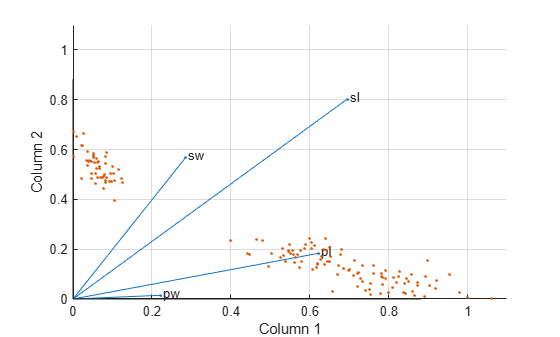# nnmf

Nonnegative matrix factorization

## Syntax

```[W,H] = nnmf(A,k) [W,H] = nnmf(A,k,param1,val1,param2,val2,...) [W,H,D] = nnmf(...) ```

## Description

`[W,H] = nnmf(A,k)` factors the nonnegative n-by-m matrix `A` into nonnegative factors `W` (n-by-`k`) and `H` (`k`-by-m). The factorization is not exact; `W*H` is a lower-rank approximation to `A`. The factors `W` and `H` are chosen to minimize the root-mean-squared residual `D` between `A` and `W*H`:

`D = norm(A-W*H,'fro')/sqrt(N*M)`

The factorization uses an iterative method starting with random initial values for `W` and `H`. Because the root-mean-squared residual `D` may have local minima, repeated factorizations may yield different `W` and `H`. Sometimes the algorithm converges to a solution of lower rank than k, which may indicate that the result is not optimal.

`W` and `H` are normalized so that the rows of `H` have unit length. The columns of `W` are ordered by decreasing length.

`[W,H] = nnmf(A,k,param1,val1,param2,val2,...)` specifies optional parameter name/value pairs from the following table.

ParameterValue
`'algorithm'`

Either `'als'` (the default) to use an alternating least-squares algorithm, or `'mult'` to use a multiplicative update algorithm.

In general, the `'als'` algorithm converges faster and more consistently. The `'mult'` algorithm is more sensitive to initial values, which makes it a good choice when using `'replicates'` to find `W` and `H` from multiple random starting values.

`'w0'`

An n-by-`k` matrix to be used as the initial value for `W`.

`'h0'`

A `k`-by-m matrix to be used as the initial value for `H`.

`'options'`

An options structure as created by the `statset` function. `nnmf` uses the following fields of the options structure:

• `Display` — Level of display. Choices:

• `'off'` (default) — No display

• `'final'` — Display final result

• `'iter'` — Iterative display of intermediate results

• `MaxIter` — Maximum number of iterations. Default is `100`. Unlike in optimization settings, reaching `MaxIter` iterations is treated as convergence.

• `TolFun` — Termination tolerance on change in size of the residual. Default is `1e-4`.

• `TolX` — Termination tolerance on relative change in the elements of `W` and `H`. Default is `1e-4`.

• `UseParallel` — Set to `true` to compute in parallel. Default is `false`.

• `UseSubstreams` — Set to `true` to compute in parallel in a reproducible fashion. Default is `false`. To compute reproducibly, set `Streams` to a type allowing substreams: `'mlfg6331_64'` or `'mrg32k3a'`.

• `Streams` — A `RandStream` object or cell array of such objects. If you do not specify `Streams`, `nnmf` uses the default stream or streams. If you choose to specify `Streams`, use a single object except in the case

• `UseParallel` is `true`

• `UseSubstreams` is `false`

In that case, use a cell array the same size as the Parallel pool.

To compute in parallel, you need Parallel Computing Toolbox™.

`'replicates'`

The number of times to repeat the factorization, using new random starting values for `W` and `H`, except at the first replication if `'w0'` and `'h0'` are given. This is most beneficial with the `'mult'` algorithm. The default is `1`.

`[W,H,D] = nnmf(...)` also returns `D`, the root mean square residual.

## Examples

collapse all

`load fisheriris`

Compute a nonnegative rank-two approximation of the measurements of the four variables in Fisher's iris data.

```rng(1) % For reproducibility [W,H] = nnmf(meas,2); H```
```H = 2×4 0.6945 0.2856 0.6220 0.2218 0.8020 0.5683 0.1834 0.0149 ```

The first and third variables in `meas` (sepal length and petal length, with coefficients 0.6945 and 0.6220, respectively) provide relatively strong weights to the first column of `W` . The first and second variables in `meas` (sepal length and sepal width, with coefficients 0.8020 and 0.5683) provide relatively strong weights to the second column of `W` .

Create a `biplot` of the data and the variables in `meas` in the column space of `W` .

```biplot(H','scores',W,'varlabels',{'sl','sw','pl','pw'}); axis([0 1.1 0 1.1]) xlabel('Column 1') ylabel('Column 2')```Starting from a random array `X` with rank 20, try a few iterations at several replicates using the multiplicative algorithm:

```X = rand(100,20)*rand(20,50); opt = statset('MaxIter',5,'Display','final'); [W0,H0] = nnmf(X,5,'replicates',10,... 'options',opt,... 'algorithm','mult');```
``` rep iteration rms resid |delta x| 1 5 0.560887 0.0245182 2 5 0.66418 0.0364471 3 5 0.609125 0.0358355 4 5 0.608894 0.0415491 5 5 0.619291 0.0455135 6 5 0.621549 0.0299965 7 5 0.640549 0.0438758 8 5 0.673015 0.0366856 9 5 0.606835 0.0318931 10 5 0.633526 0.0319591 Final root mean square residual = 0.560887 ```

Continue with more iterations from the best of these results using alternating least squares:

```opt = statset('Maxiter',1000,'Display','final'); [W,H] = nnmf(X,5,'w0',W0,'h0',H0,... 'options',opt,... 'algorithm','als');```
``` rep iteration rms resid |delta x| 1 24 0.257336 0.00271859 Final root mean square residual = 0.257336 ```

## References

 Berry, M. W., et al. “Algorithms and Applications for Approximate Nonnegative Matrix Factorization.” Computational Statistics and Data Analysis. Vol. 52, No. 1, 2007, pp. 155–173.